A challenge for statistical programmers is getting data into the right form for analysis. For graphing or analyzing data, sometimes the "wide format" (each subject is represented by one row and many variables) is required, but other times the "long format" (observations for each subject span multiple rows) is more useful. I've previously written about how to reshape data from wide to long format.

It can be a challenge to get data in the right shape. For years I have wrestled with reformatting output data sets when computing descriptive statistics for multiple variables. One of the powers of SAS software is that you can specify many variables in a procedure (such as MEANS or UNIVARIATE) and the procedure computes the requested statistics for each variable. However, to use that output in a subsequent graph or analysis sometimes requires reshaping the output data set.

Since it is inconvenient to have to reshape data sets, this article describes three ways to produce descriptive statistics (in particular, percentiles) in a tabular form in which variables form columns (or rows) and the statistics are arranged in the other dimension. This is usually the easiest shape to work with.

### For standard percentiles, use PROC MEANS

As a canonical example, consider the the task of computing multiple percentiles for several variables when the underlying data are in a wide format. By default, both PROC MEANS and PROC UNIVARIATE create the output data set in a less-than-optimal shape.

For commonly used percentiles (such as the 5th, 25th, 50th, 75th, and 95th percentiles), you can use PROC MEANS and the STACKODSOUTPUT option, which was introduced in SAS 9.3, to create an output data set that contains percentiles for the multiple variables in a more convenient format. Compare the following two output data sets. The first output is usually harder to work with than the second:

```/* default output data set. Yuck! */ proc means data=sashelp.cars noprint; var mpg_city mpg_highway; output out=MeansWidePctls P5= P25= P75= P95= / autoname; run;   proc print data=MeansWidePctls noobs; run;``````/* Use the STACKODSOUTPUT option to get output in a more natural shape */ proc means data=sashelp.cars StackODSOutput P5 P25 P75 P95; var mpg_city mpg_highway; ods output summary=LongPctls; run;   proc print data=MeansLongPctls noobs;run;```If I want to use the percentiles in a subsequent analysis, such as plotting the percentiles on a graph, I much prefer to work with the second set of output.

### For nonstandard percentiles, use PROC STDIZE

For many years I have used PROC UNIVARIATE to compute custom percentiles. One application of custom percentiles is to compute a 95% bootstrap confidence interval, which requires computing the 2.5th and 97.5th percentiles. However, when you are analyzing multiple variables PROC UNIVARIATE creates an output data set that is a single row, as shown in the following example:

```/* default output data set. Yuck! */ proc univariate data=sashelp.cars noprint; var mpg_city mpg_highway; output out=UniWidePctls pctlpre=CityP_ HwyP_ pctlpts=2.5,15,65,97.5; run;   proc print data=UniWidePctls noobs; run;```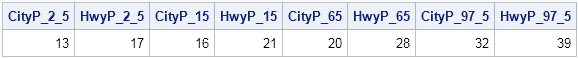There are many tricks, papers, and macros published that reshape or restructure the output from PROC UNIVARIATE. In my book, Simulating Data with SAS, I show one technique for reshaping the output. (If you have a favorite method, link to it in the comment section.)

And so it was a surprise to find out that the easiest way to get customized percentiles in a tabular form is to not use PROC UNIVARIATE! It turns out that the STDIZE procedure also computes custom percentiles, and the output is written in a tabular form. I knew that PROC STDIZE standardizes variables, but I didn't know that it computes custom percentiles.

The PROC STDIZE syntax is easy and very similar to the PROC UNIVARIATE syntax: use the PCTLPTS= option to specify the custom percentiles. Unlike PROC UNIVARIATE, you do not need PCTLPRE= option, because the names of the variables are used for the output variables, and the percentiles are just additional rows that are added to the output data set. A typical example follows:

```proc stdize data=sashelp.cars PctlMtd=ORD_STAT outstat=StdLongPctls pctlpts=2.5,15,65,97.5; var mpg_city mpg_highway; run;   proc print data=StdLongPctls noobs; where _type_ =: 'P'; run;```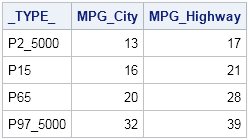I explicitly specified the PCTLMTD= option so that the algorithm uses the traditional "sort the data" algorithm for computing percentiles, rather than a newer one-pass algorithm. Although the one-pass algorithm is very fast and well-suited for computing the median, it is not recommended for computing extreme percentiles such as the 2.5th and 97.5th.

### Or use the SAS/IML language

Of course, no blog post from me is complete without showing how to compute the same quantity by using the SAS/IML language. The QNTL subroutine computes customized quantiles. (Recall that quantiles and percentiles are essentially the same: The 0.05 quantile is the 5th percentile, the 0.25 quantile is the 25th percentile, and so forth.) By using the QNTL subroutine, the quantiles automatically are packed into a matrix where each column corresponds to a variable and each row corresponds to a quantile, as follows:

```proc iml; varNames = {"mpg_city" "mpg_highway"}; use sashelp.cars; read all var varNames into X; close sashelp.cars;   Prob = {2.5, 15, 65, 97.5} / 100; /* prob in (0,1) */ call qntl(Pctls, X, Prob); print Prob Pctls[c=varNames];```So there you have it. Three ways to compute percentiles (quantiles) so that the results are shaped in a tabular form. For standard percentiles, use PROC MEANS with the STACKODSOUTPUT option. For arbitrary percentiles, use PROC STDIZE or PROC IML. If you use these techniques, the percentiles are arranged in a tabular form and you do not need to run any additional macro or DATA step to reshape the output.On Kaiser Fung's Junk Charts blog, he showed a bar chart that was "published by Teach for America, touting its diversity." Kaiser objected to the chart because the bar lengths did not accurately depict the proportions of the Teach for America corps members.

The chart bothers me for another reason: I think if you create a graph that purports to demonstrate racial diversity, your graph should provide as reference the national percentages of each racial group. In general, if you claim that your organization does something better than a benchmark, you should include the benchmark values. (For mutual fund companies, comparing performance to a benchmark index is not just a good idea, it is the law.)

It turns out that the U.S. Census Bureau does not count "Latino" as a racial or ethnic group, but I used data and information from the 2010 US census to estimate the racial distribution of the US population. The following data and graphs compare the diversity of Teach for America to the national percentages:

```title "Teach for America Diversity versus US Average"; data TeachAmerica; input Race \$28. Corps USPop; label Diff="Difference between Corps and US Percentages" RelDiff="Relative Difference between Corps and US"; Diff = Corps - USPop; /* O-E = observed - expected */ RelDiff = Diff / USPop; /* relative diff: (O-E)/E */ datalines; Causasian 62 56 African American 13 13 Latino 10 16 Asian American 6 5 Multi-Ethnic 5 6 Other 4 3 Native American or Hawaiian 0.5 1.1 ;   proc sgplot data=TeachAmerica; /* standard dot plot with reference */ scatter y=Race x=USPop / markerattrs=(symbol=Diamond) legendlabel="US"; scatter y=Race x=Corps / markerattrs=(symbol=CircleFilled) legendlabel="Corps"; yaxis grid; run;   proc sgplot data=TeachAmerica; /* plot differences from US population */ scatter y=Race x=Diff / markerattrs=(symbol=CircleFilled); refline 0 / axis=x; yaxis grid; run;```

Two graphs are created, but only one is shown. The first graph (not shown) is a dot plot of the Teach for America percentages overlaid on a dot plot of the US percentages for each race. This essentially reproduces the Teach for America bar chart, but adds the reference percentages. I use a dot plot instead of a bar chart so that the reference percentages are easier to see.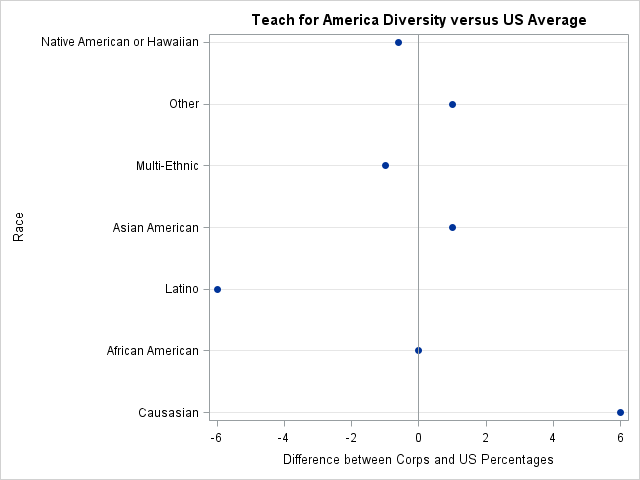However, as I've said recently, when you are comparing two groups, you should strongly consider creating a graph of the difference between the two groups. The differences are shown in the adjacent figure. (Click to enlarge.) The graph shows that there is not much difference between the diversity of Teach for America and the US population. Five of the seven racial groups are within a percentage point of the national average. Latinos are underrepresented in the Teach for America corps, whereas Caucasians are overrepresented.

The graph tells a more complete story than the Teach for America article, which claims "a higher level of diversity in our corps" than US colleges. Although the Teach for America corps is not overrepresented in all minority groups compared to the US population, they should be commended for closely matching the diversity of the US population. The graph also sends a message: although Teach for America has done a great job with diversity, they need to continue to work on recruiting Latino teachers.

When visualizing differences, sometimes it is useful to compute relative differences. Caucasians are a large ethnic group, so although a deviation of 6 percentage points is large in absolute terms, it is small in relative terms. To create this graph by using PROG SGPLOT, specify the RelDiff variable instead of the Diff variable.

It is worth noting that SAS software makes it easy to graph and analyze data like these. The TABLES statement in the FREQ procedure supports a TESTP= option that enables you to specify proportions for a chi-square test for proportions. You can also request a DeviationPlot, which shows the relative differences between the observed proportions and the specified proportions. To use PROC FREQ, you should convert the percentages into frequencies. The Teach for America Web site says that there were 11,000 teachers in the program in 2013, so I will use that number to convert the percentages into frequencies.

```data Teach; set TeachAmerica; N = 11000 * Corps / 100; /* approx number of people in corps for each group */ run;   proc freq data=Teach order=data; weight N; tables Race / chisq testp=(.56 .13 .16 .05 .06 .03 .011) plots(type=dotplot)=(DeviationPlot FreqPlot(scale=percent)); run;```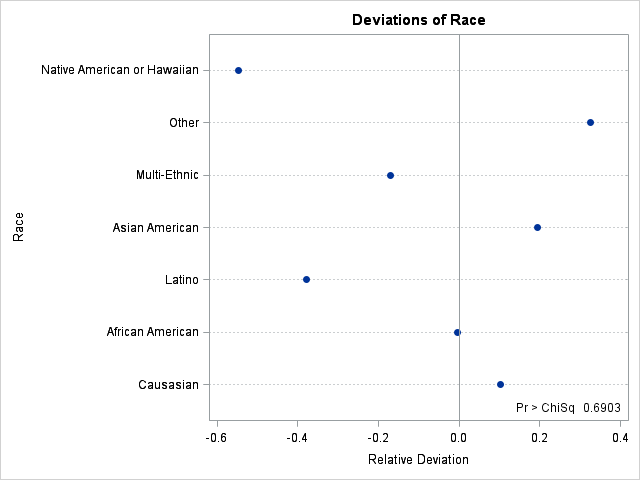The graph shows that the Native American and Latino groups are underrepresented on a relative basis, whereas the "Other" group is overrepresented. On a relative scale, Caucasians are only slightly overrepresented. The chi-square table (not shown) indicates that Teach for America proportions are not equal to the national proportions. The chi-square p-value is tiny, and is included in the lower right corner of the graph.

In summary, if you claim that certain quantities are as good as or better than some benchmark, include the benchmark as part of your graph. Even better, graph the difference between your quantities and the benchmark. SAS software provides tools to construct the graphs by hand, or as part of a statistical test for proportions.In my last blog post I described how to implement a "runs test" in the SAS/IML language. The runs test determines whether a sequence of two values (for example, heads and tails) is likely to have been generated by random chance. This article describes two applications of the runs test. In both cases, you have to recode the data into a two-value sequence in order to use the runs test. The applications are:

• Application 1: Are two samples from the same population?
• Application 2: Given a series of univariate numerical values, is there evidence that the data are random deviations from a constant value?

### Are two samples from the same population?

The runs test can be applied to the problem of determining whether two samples are from the same population. If you measure some quantity for two groups, you might want to know whether the distribution of that quantity is the same for both groups.

There are various parametric tests that test whether some parameter is the same in both groups. In SAS software, you can use the TTEST procedure to test whether two samples are from the same normal populations. If you do not want to make a parametric assumption on the distribution of the population, there are many distribution-free tests in the NPAR1WAY procedure. The runs test is not included in PROC NPAR1WAY because there are other tests that have greater statistical power. (In fact, Jame Bradley's Distribution-Free Statistical Tests (1968, p. 263) says "This is one of the least powerful distribution-free tests.")

Nevertheless, let's see how you can use the runs test to test for identical populations. The Sashelp.bweight data set contains the birth weight of 50,000 babies. The following DATA step extracts the first 50 observations from the data: 31 boys and 19 girls. You might want to know whether the distribution of birth weight for boys is the same as the distribution of weight for the girls.

```data bweight; /* choose 50 obs subset of the data */ set sashelp.bweight(keep=Weight Boy); N = _N_; if _N_<=50; run;   proc sgplot data=bweight; title "Birth weight of 50 Babies"; scatter x=N y=Weight / group=Boy markerattrs=(symbol=CircleFilled); keylegend / position=bottomright location=inside across=1 title="Boy"; xaxistable boy / location=inside; /* SAS 9.4 feature */ run;```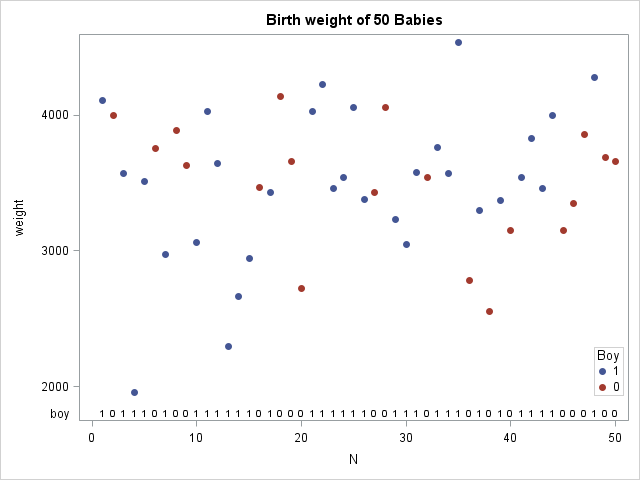The data are shown in the scatter plot, which also demonstrates a cool new feature of SAS 9.4. The XAXISTABLE statement is used to display a small table at the bottom of the graph that indicates whether each observation is a boy (1) or girl (0)! (Click to enlarge.)

If the two samples (weights of boys and girls) come from the same population, the boys and girls should be randomly distributed in a sequence of the ordered weights. However, if girls tend to weigh less than boys, you would expect to see more girls at the beginning of the sequence and more boys at the end. You can use the runs test to determine whether the sequence is random.

The following SAS/IML program reads the weight and gender of the babies. The program sorts the data by weight and uses the runs test to analyze the resulting sequence of genders. The RunsTest module needs to be defined or loaded before it is called.

```proc iml; load module=RunsTest; /* load or define the RunsTest module */   /* Application 1: Determine whether two samples are from the same population. Sort the data. Form two-value sequence that indicates the sample to which it belongs. If populations are identical, the sequence is random. */ use bweight; read all var {"Weight" "Boy"} into X; close bweight;   call sort(X, 1); /* sort data by weight */ seq = X[,2]; /* two-value sequence to test */ test = RunsTest(seq); print test[c={"Runs Statistic" "p-value"} L="Runs Test (Two Populations)"];```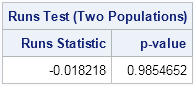The RunsTest module returns a large p-value, which indicates that the sequence appears to be random. Notice that this is a distribution-free test because it uses only the fact that the data are from two groups. The test does not assume any special shape for the distribution of the population.

### Is a sequence randomly distributed about some central value?

Consider the problem of filling a box with cereal as it rolls down an assembly line. If the filling process is in control, the amount of cereal in each box is the target value (such as 450 grams) plus or minus some small random amount. If you weigh a sequence of cereal boxes, you can divide the units into two categories: those that weigh more than the target value and those that weigh less. If the variation is random, the sequence of "More" and "Less" should be random.

By subtracting the target value from each box's weight, the sequence is transformed into a sequence of positive and negative values. The following SAS/IML statements model the assembly line by simulating a sequence of 30 random values from a standard normal distribution. Positive values are assigned the value +1 and negative values are assigned the value –1. The new sequence of values {+1, –1, –1,..., +1} is sent to the RunsTest module:

```/* Application 2: Is P(X<=0) constant during data generation? */ call randseed(123); x = j(30,1); /* 30 x 1 vector */ call randgen(x, "Normal"); /* fill with random normal values */ s = sign(x); /* s=1 iff x>0 */ Test = RunsTest(s); print Test[c={"Runs Statistic" "p-value"} L="Runs Test (Normal Sequence)"];```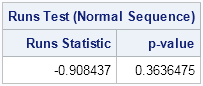The runs test returns a large p-value, which indicates that there is no reason to doubt that the sequence is random. Although this does not prove that the RANDGEN function generates random values, it does indicate that there is no pattern to the positive and negative values.

Although the runs test is not very powerful, the test is simple and easy to understand, and rather fun to think about and implement. It is also a good example for showing how you can use the SAS/IML language to extend the statistical capabilities of SAS software.

tags: Data AnalysisIn my last blog post I described how to implement a "runs test" in the SAS/IML language. The runs test determines whether a sequence of two values (for example, heads and tails) is likely to have been generated by random chance. This article describes two applications of the runs test. In both cases, you have to recode the data into a two-value sequence in order to use the runs test. The applications are:

• Application 1: Are two samples from the same population?
• Application 2: Given a series of univariate numerical values, is there evidence that the data are random deviations from a constant value?

### Are two samples from the same population?

The runs test can be applied to the problem of determining whether two samples are from the same population. If you measure some quantity for two groups, you might want to know whether the distribution of that quantity is the same for both groups.

There are various parametric tests that test whether some parameter is the same in both groups. In SAS software, you can use the TTEST procedure to test whether two samples are from the same normal populations. If you do not want to make a parametric assumption on the distribution of the population, there are many distribution-free tests in the NPAR1WAY procedure. The runs test is not included in PROC NPAR1WAY because there are other tests that have greater statistical power. (In fact, Jame Bradley's Distribution-Free Statistical Tests (1968, p. 263) says "This is one of the least powerful distribution-free tests.")

Nevertheless, let's see how you can use the runs test to test for identical populations. The Sashelp.bweight data set contains the birth weight of 50,000 babies. The following DATA step extracts the first 50 observations from the data: 31 boys and 19 girls. You might want to know whether the distribution of birth weight for boys is the same as the distribution of weight for the girls.

```data bweight; /* choose 50 obs subset of the data */ set sashelp.bweight(keep=Weight Boy); N = _N_; if _N_<=50; run;   proc sgplot data=bweight; title "Birth weight of 50 Babies"; scatter x=N y=Weight / group=Boy markerattrs=(symbol=CircleFilled); keylegend / position=bottomright location=inside across=1 title="Boy"; xaxistable boy / location=inside; /* SAS 9.4 feature */ run;```The data are shown in the scatter plot, which also demonstrates a cool new feature of SAS 9.4. The XAXISTABLE statement is used to display a small table at the bottom of the graph that indicates whether each observation is a boy (1) or girl (0)! (Click to enlarge.)

If the two samples (weights of boys and girls) come from the same population, the boys and girls should be randomly distributed in a sequence of the ordered weights. However, if girls tend to weigh less than boys, you would expect to see more girls at the beginning of the sequence and more boys at the end. You can use the runs test to determine whether the sequence is random.

The following SAS/IML program reads the weight and gender of the babies. The program sorts the data by weight and uses the runs test to analyze the resulting sequence of genders. The RunsTest module needs to be defined or loaded before it is called.

```proc iml; load module=RunsTest; /* load or define the RunsTest module */   /* Application 1: Determine whether two samples are from the same population. Sort the data. Form two-value sequence that indicates the sample to which it belongs. If populations are identical, the sequence is random. */ use bweight; read all var {"Weight" "Boy"} into X; close bweight;   call sort(X, 1); /* sort data by weight */ seq = X[,2]; /* two-value sequence to test */ test = RunsTest(seq); print test[c={"Runs Statistic" "p-value"} L="Runs Test (Two Populations)"];```The RunsTest module returns a large p-value, which indicates that the sequence appears to be random. Notice that this is a distribution-free test because it uses only the fact that the data are from two groups. The test does not assume any special shape for the distribution of the population.

### Is a sequence randomly distributed about some central value?

Consider the problem of filling a box with cereal as it rolls down an assembly line. If the filling process is in control, the amount of cereal in each box is the target value (such as 450 grams) plus or minus some small random amount. If you weigh a sequence of cereal boxes, you can divide the units into two categories: those that weigh more than the target value and those that weigh less. If the variation is random, the sequence of "More" and "Less" should be random.

By subtracting the target value from each box's weight, the sequence is transformed into a sequence of positive and negative values. The following SAS/IML statements model the assembly line by simulating a sequence of 30 random values from a standard normal distribution. Positive values are assigned the value +1 and negative values are assigned the value –1. The new sequence of values {+1, –1, –1,..., +1} is sent to the RunsTest module:

```/* Application 2: Is P(X<=0) constant during data generation? */ call randseed(123); x = j(30,1); /* 30 x 1 vector */ call randgen(x, "Normal"); /* fill with random normal values */ s = sign(x); /* s=1 iff x>0 */ Test = RunsTest(s); print Test[c={"Runs Statistic" "p-value"} L="Runs Test (Normal Sequence)"];```The runs test returns a large p-value, which indicates that there is no reason to doubt that the sequence is random. Although this does not prove that the RANDGEN function generates random values, it does indicate that there is no pattern to the positive and negative values.

Although the runs test is not very powerful, the test is simple and easy to understand, and rather fun to think about and implement. It is also a good example for showing how you can use the SAS/IML language to extend the statistical capabilities of SAS software.

tags: Data AnalysisWhile walking in the woods, a statistician named Goldilocks wanders into a cottage and discovers three bears. The bears, being hungry, threaten to eat the young lady, but Goldilocks begs them to give her a chance to win her freedom.

The bears agree. While Mama Bear and Papa Bear block Goldilocks' view, Baby Bear tosses a coin 30 times and records the results. He then makes up two other (fake) sequences of heads and tails, and gives Goldilocks a piece of paper that shows all three sequences. Papa Bear growls, "If you can determine which sequence came from the real coin toss, we will let you go. Otherwise we will eat you for dinner, for I have grown tired of porridge."

Here are the three sequences of heads (H) and tails (T) that the bears present to Goldilocks. Each of the sequences contain 16 heads and 14 tails.

1. H H H H H H H H H H H H H H H H T T T T T T T T T T T T T T
2. H T H T H T H T H T H T H T H T H T H T H T H T H T H T H H
3. H T T H H H T T T T T T T H H H T H T H H H T H H H T H T H

Goldilocks studies the three sequences and tells Papa Bear:

The first sequence is "too hot." It contains 16 heads followed by 14 tails. I would not expect such long sequences of heads and tails. Similarly, the second sequence is "too cold." It alternates between heads and tails like clockwork. The third sequence is "just right." It matches my intuitive notion of a random sequence of two categories: many short subsequences interlaced with a few longer subsequences. I think that the third sequence is real.

She had chosen correctly. The three bears, impressed by her statistical knowledge, set Goldilocks free and—once again—reluctantly ate porridge for dinner.

### Implementing the "runs test" in SAS

Goldilocks reasoned well. If she had brought along her laptop with SAS software on it, she could have used statistics to analyze the data.

You can quantify Goldilocks' intuitive notions by defining a run as a sequence of consecutive trials that result in the same value. The first sequence has two runs: a run of heads followed by a run of tails. The second sequence has 29 runs. The third sequence has 15 runs: eight runs of heads and seven runs of tails.

It turns out that you can calculate the expected number of runs in a random sequence that has n heads and m tails. The expected number of runs is E(R) = 2nm / (n+m) + 1. The three sequences have n=16 heads and m=14 tails, so the expected number of runs is 15.9. So Goldilocks' intuition was correct: the first sequence does not have enough runs, whereas the second has too many. The third sequence has 15 runs, which is close to the expected value.

You can use these facts to construct a statistical test for the randomness of a two-category sequence, based on the number of runs in the sequence. This is called the runs test, or the Wald-Wolfowitz test for randomness. There is a SAS Knowledge Base article that describes how to implement the runs test in SAS by using the DATA step. However, the DATA step implementation is longer and more complicated than an analogous implementation in the SAS/IML language.

To simplify counting the number of runs, recode the two categories (heads and tails) as –1 and +1. Then a "run" is a sequence of consecutive values that have the same sign. I have previously blogged about how to use the DIF-SIGN technique to detect when terms in a sequence switch signs. In the following program, that technique is used to count the number of runs in a sequence.

```proc iml; /* Implement the Wald-Wolfowitz test (or "runs test"). The SEQ vector is assumed to contain two groups (char or numeric). Compare with Base SAS version: http://support.sas.com/kb/33/092.html */ start RunsTest(seq); u = unique(seq); /* find unique values (should be two) */ d = j(nrow(seq)*ncol(seq),1, 1);/* recode as vector of -1, +1 */ d[loc(seq=u)] = -1; n = sum(d>0); /* count number of +1s */ m = sum(d<0); /* count number of -1s */   /* Use DIF-SIGN trick to count the number of runs */ dif = dif(sign(d)); /* difference in signs */ dif = choose(d<0, -2, 2); /* replace first obs */ R = sum(dif=2 | dif=-2); /* R = observed number of runs */   mu = 2*n*m / (n+m) + 1; /* E(R)=expected number of runs, given n, m */ var = 2*n*m*(2*n*m-(n+m)) / ((n+m)##2*(n+m-1)); /* var(R) */ sigma = sqrt(var); /* StdDev(R) */   /* R is asymptotically normally distributed. Compute test statistic. */ if (n+m)>50 then Z = (R-mu) /sigma; else if R-mu < 0 then Z = (R-mu+0.5)/sigma; /* for small samples, apply */ else Z = (R-mu-0.5)/sigma; /* continuity correction */   return(Z || 2*(1-cdf("Normal", abs(Z)))); /* (Z, two-sided p-value) */ finish;   flips = {H T T H H H T T T T T T T H H H T H T H H H T H H H T H T H}`; Test = RunsTest(flips); print Test[c={"Runs Statistic" "p-value"} L="Runs Test, two-sided"];```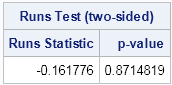The output of the RunsTest module is a two-element vector. The first element is the test statistic and the second element is the two-side p-value. Running the test on Baby Bear's coin-toss sequence produces a large p-value, which means that Goldilocks would accept the null hypothesis that the sequence of coin tosses is random. You can run the test for the other two sequences ("too hot" and "too cold") and see that both of them produce tiny p-values. If Goldilocks had implemented the runs test, she would have concluded that the made-up sequences are unlikely to be random sequence of coin flips.

I'll conclude with a few thoughts:

• The SAS/IML language is useful for implementing statistical methods, such as the runs test, that are not included in any SAS procedure.
• The SAS/IML language is compact. For this example, the SAS/IML program contains about 15 lines, versus 65 lines for the DATA step code.
• When I first thought about this problem, I thought I would need to use a loop to count the number of runs in a sequence. However, by recoding the data as +/–1, I was able to vectorize the computation by using the DIF-SIGN technique.
• Goldilocks was fortunate to know statistics."It's a floor wax, and a dessert topping" - this pretty much describes SAS/Graph! (bonus points if you know where this quote came from!) Some people think of SAS as just a quality control tool. Others think of it as just a sales & marketing tool. And yet others think [...]SAS has supported calling R from the SAS/IML language since 2009. The interface to R is part of the SAS/IML language. However, there have been so many versions of SAS and R since 2009, that it is hard to remember which SAS release supports which versions of R. The following table lists recent SAS releases and the versions of R that each supports: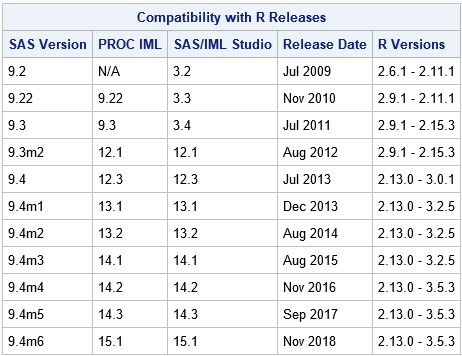There were two releases of R that broke backwards compatibility with prior SAS releases:

• R 2.12.0, which introduced 64-bit R, changed the locations of certain libraries. To use R 2.12.0 or later, you must use SAS 9.3 or later.
• R 3.0.0 changed certain aspects of the external API to R. To use R 3.0.0 or later you must use SAS 9.4 or later.
An error message that you might see because of incompatible versions is "An installed version of R could not be found," although this message can also occur for other reasons.

SAS ensures that each SAS release supports backwards compatibility with as many previous R releases as possible. However, after a version of SAS ships, it is impossible to ensure forward compatibility, as evidenced by the R 3.0.0 release. That version of R was released in April 2013; it is supported in SAS 9.4 software, which shipped a few months later.

Some software companies distribute their own versions of R, but SAS does not. Consequently, if the interface to R changes, SAS customers need to use a compatible version of R until they can upgrade to a more recent version of SAS. To reiterate:

• If you are running a version of SAS prior to SAS 9.4, you should use R 2.15.x or earlier.
• You can call R 3.0.x by using SAS/IML 12.3 or later. SAS/IML 12.3 shipped in July 2013 as part of SAS 9.4.

I'll close with a few comments about 32-bit and 64-bit versions of SAS and R.

• If you are using SAS software on a 32-bit edition of Windows, you must install the 32-bit edition of R.
• If you are using SAS software on a 64-bit edition of Windows, you can install either the 32-bit or the 64-bit edition of R. The 32-bit edition of SAS looks first for the 32-bit edition of R and then for the 64-bit edition. The 64-bit edition of SAS looks first for the 64-bit edition of R and then for the 32-bit edition.SAS has supported calling R from the SAS/IML language since 2009. The interface to R is part of the SAS/IML language. However, there have been so many versions of SAS and R since 2009, that it is hard to remember which SAS release supports which versions of R. The following table lists recent SAS releases and the versions of R that each supports:To date, three releases of R broke backwards compatibility with prior SAS releases:

• R 2.12.0, which introduced 64-bit R, changed the locations of certain libraries. To use R 2.12.0 or later, you must use SAS 9.3 or later.
• R 3.0.0 changed certain aspects of the external API to R. To use R 3.0.0 or later you must use SAS 9.4 or later.
• R 3.0.2 changed an internal detail that SAS was using. SAS/IML 13.1 will support R 3.0.2.
An error message that you might see because of incompatible versions is "An installed version of R could not be found," although this message can also occur for other reasons.

SAS ensures that each SAS release supports backwards compatibility with as many previous R releases as possible. However, after a version of SAS ships, it is impossible to ensure forward compatibility, as evidenced by the R 3.0.0 release. That version of R was released in April 2013; it is supported in SAS 9.4 software, which shipped a few months later.

Some software companies distribute their own versions of R, but SAS does not. Consequently, if the interface to R changes, SAS customers need to use a compatible version of R until they can upgrade to a more recent version of SAS. To reiterate:

• If you are running a version of SAS prior to SAS 9.4, you should use R 2.15.x or earlier.
• You can call R 3.0.1 by using SAS/IML 12.3 or later. SAS/IML 12.3 shipped in July 2013 as part of SAS 9.4.
• You can call R 3.0.2 and later by using SAS/IML 13.1 or later. SAS/IML 13.1 is scheduled to ship in December 2013 as part of SAS 9.4m1.

I'll close with a few comments about 32-bit and 64-bit versions of SAS and R.

• If you are using SAS software on a 32-bit edition of Windows, you must install the 32-bit edition of R.
• If you are using SAS software on a 64-bit edition of Windows, you can install either the 32-bit or the 64-bit edition of R. The 32-bit edition of SAS looks first for the 32-bit edition of R and then for the 64-bit edition. The 64-bit edition of SAS looks first for the 64-bit edition of R and then for the 32-bit edition.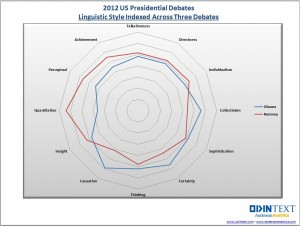A common visualization is to compare characteristics of two groups. This article emphasizes two tips that will help make the comparison clear. First, consider graphing the differences between the groups. Second, in any plot that has a categorical axis, sort the categories by a meaningful quantity.

This article is motivated by a radar plot that compares the performance of Barak Obama and Mitt Romney in the 2012 US presidential debates. The radar plot (shown at left) displays 12 characteristics of the candidates' words during the debates. Characteristics included "directness", "talkativeness", and "sophistication." The radar chart accompanies an interesting article about using text analytics to investigate the candidates' styles and performance during the debates.

Unfortunately, I found it difficult to use the radar chart to compare the candidates. I wondered whether a simpler chart might be more effective. I couldn't find the exact numbers used to create the radar chart, but I could use the radar chart to estimate the values. The following DATA step defines the data. The numbers seem to be the relative percentages that each candidate displayed a given characteristic. (Possibly weighted by the amount of time that each candidate spoke?) Each row adds to 100%. For example, of the words and phrases that were classified as exhibiting "individualism," 47% of the phrases were uttered by Obama, and 53% by Romney.

```data Debate2012; input Category \$15. Obama Romney; datalines; Individualism 47 53 Directness 49 51 Talkativeness 49 51 Achievement 45 55 Perceptual 44 56 Quantitative 36 64 Insight 47 53 Causation 59 41 Thinking 52 48 Certainty 56 44 Sophistication 51 49 Collectivism 56 44 ;```

You can use the data in this form to display a series plot (line plot) of the percentages versus the categories, but the main visual impact of the line plot is that Obama's values are low when Romney's are high, and vice versa. Since that is just a consequence of the fact that the percentages add to 100%, I do not display the series plot.

To display a bar chart, you can create a grouping variable with the values "Obama" and "Romney." You can then use the GROUP= option on the HBAR statement in PROC SGPLOT to create the following stacked bar chart:

```data Long; /* transpose the data from wide to long */ set Debate2012; Candidate = "Obama "; Value = Obama; output; Candidate = "Romney"; Value = Romney; output; drop Obama Romney; run;   title "2012 US Presidential Debates"; title2 "Linguistic Style Indexed Across Three Debates"; footnote justify=left "Data from http://blog.odintext.com/?p=179"; proc sgplot data=Long; hbar Category / response=Value group=Candidate groupdisplay=stack; refline 50 / axis=x lineattrs=(color=black); yaxis discreteorder=data; xaxis label="Percentage" values=(0 to 100 by 10); run;```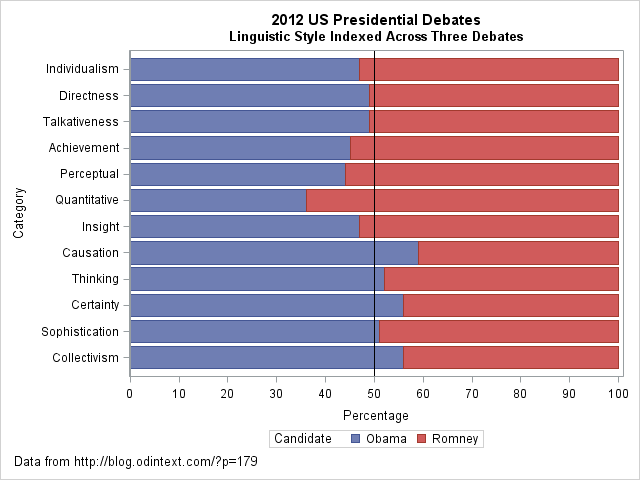The stacked bar chart makes it easy to compare the styles of the two candidates. In some categories (directness, talkativeness, and sophistication), the candidates are similar. In others (quantitative, causation), the candidates differ more dramatically. A prominent reference line at 50% provides a useful dividing line for comparing the candidates' styles.

However, I see two problems with the stacked bar chart. First, the categories do not seem to be sorted in any useful order. (This order was used for the radar chart.) Second, I often find that if you want to contrast two groups, it often makes sense to plot the difference between the groups, rather than the absolute quantities. This results in the following changes:
• The reference line becomes zero, which represents no difference.
• The chart can use a smaller scale, so that it is easier to see small differences.
• You can sort the categories by the difference, so that the order of the categories becomes useful.
• The difference is a single quantity, which is easier to visualize than two categories. (Although, since the percentages sum to 100%, there is only one independent quantity no matter how you visualize the data.)

The following DATA step uses the original data to compute the percentage difference between the candidates for each style category. Although it is not necessary to use two colors to visualize a single quantity, in US politics it is a convention to use red to represent Republicans (Romney) and blue to represent Democrats (Obama).

```data DebateDiff; set Debate2012; label Difference = "Percentage Difference: Romney - Obama"; Difference = Romney - Obama; if Difference>0 then Advantage = "Romney"; else Advantage = "Obama "; run;   proc sort data=DebateDiff; /* sort categories by difference */ by Difference; run;   title2 "Linguistic Style Differences Indexed Across Three Debates"; proc sgplot data=DebateDiff; hbar Category / response=Difference group=Advantage; refline 0 / axis=x; yaxis discreteorder=data; xaxis grid; keylegend / position=topright location=inside title="Candidate" across=1; run;```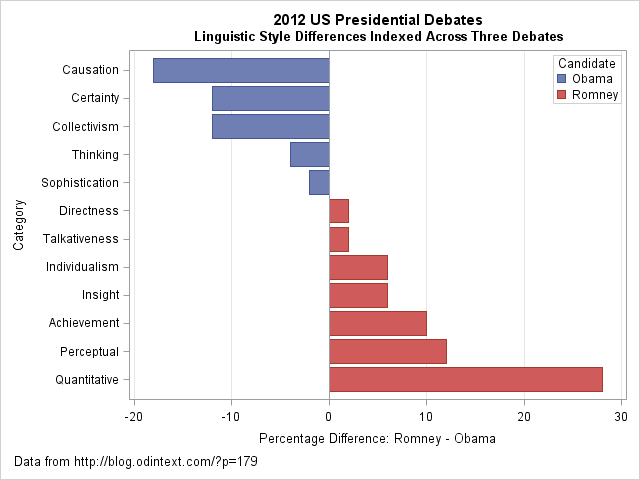I think that the "difference plot" is superior to the original radar chart and to the stacked bar chart. It makes a clear contrast of the candidates' styles. You can clearly see that Obama's words evoked causation, certainty, and collectivism much more than Romney's did. In contrast, Romney's words were perceptual and quantitative more often than Obama's. In a statistical analysis that includes uncertainties, you could even include a margin of error on this chart. For example, it might be the case that the candidates' styles were not significantly different for the sophistication, directness, and talkativeness categories. If so, those bars might be colored white or grey.

Although radar charts are useful for plotting periodic categories such as months and wind directions, I think a simple bar chart is more effective for this analysis. The main idea that I want to convey is that if you want to contrast two groups, consider displaying the difference between the groups. Also, order categories in a meaningful way, rather than default to an arbitrary order such as alphabetical order. By using these two simple tips, you can create a graph that makes a clear comparison between two groups.

What do you think? For these data, which plot would you choose to display differences between the candidates?Earlier this week I posted a "guest blog" in which my 8th grade son described a visualization of data for the 2013 ASA Poster Competition. The purpose of today's blog post is to present a higher-level statistical analysis of the same data. I will use a t test and a regression analysis with a classification variable—two concepts that are not generally known to elementary school students. (If you like analyzing kids' experiments with SAS, see also Chris Hemedinger's 2011 analysis of his daughter's "Mentos and Coke" science fair project.)

The experiment measured the drying times for 40 loads of laundry. Each load was weighed upon emerging from the washing machine. For 20 loads, my son inserted two plastic "dryer balls" into the dryer with the laundry. The other 20 loads were dried without using dryer balls. Consequently, the variables are as follows:

• Weight: the weight, in grams, of the wet clothes
• Time: the time, in minutes, required to dry the clothes, as measured by an automatic "dampness sensor" in the dryer
• Balls: an indicator variable that has the value 1 if dryer balls were included in the dryer, and 0 otherwise

### A t-test analysis

On average, does the presence of dryer balls result in shorter drying times? One way to answer this question is to ask whether the mean drying time is the same for the dryer-ball group as compared to the control loads:

```proc ttest data=DryerBalls; class Balls; var Time; run;```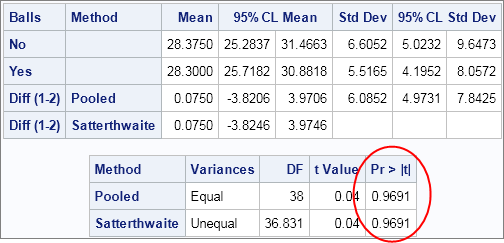The comparative histograms and side-by-side box plots indicate that the distributions of drying times are similar for the two groups. Notice that the 95% confidence interval for the difference of the means includes 0. A t test produces a large p-value (circled), which indicates that the means are not significantly different.

### A regression analysis

How does the drying time depend on the weight of the wet clothes and on the presence or absence of dryer balls? You can use the GLM procedure to model the relationship:

```ods html style=htmlbluecml; proc glm data=DryerBalls plots=all; class Balls; model Time = Weight Balls / solution; run;```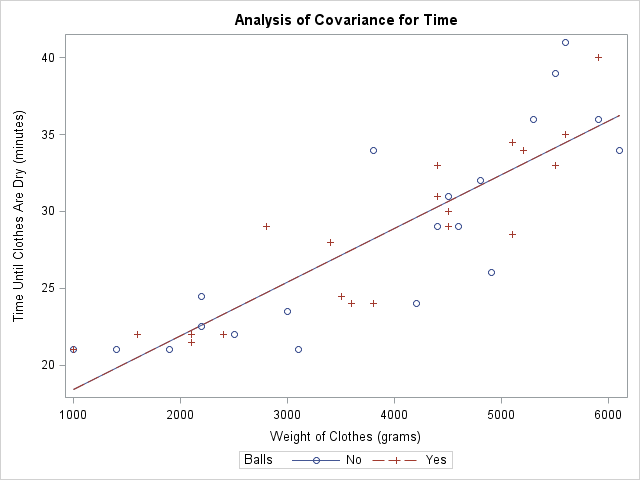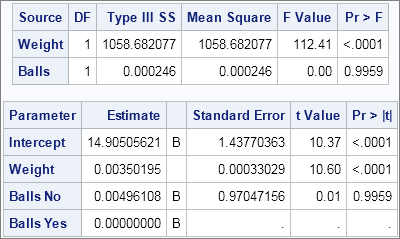The fit plot tells the story. There are actually two lines in the plot, one for Balls=1 and the other for Balls=0. However, the lines are right on top of each other, so it looks like there is only one line. The type III sum of squares and the parameter estimates confirm that the Balls variable is not significant to the model. In other words, the presence or absence of dryer balls does not significantly affect the model for drying time when controlling for the weight of the clothes. The parameter estimates indicate that each additional kilogram of clothes adds about 3.5 minutes to the drying time.

I also fit a model that includes a quadratic term for the Weight variable, but the conclusion is the same: dryer balls do not affect drying time.

I am pleased that these more sophisticated statistical analyses give the same conclusions as my son observed while using more elementary methods. Can you think of additional analyses that would be appropriate? Download the data and let me know what you discover.

tags: Data Analysis